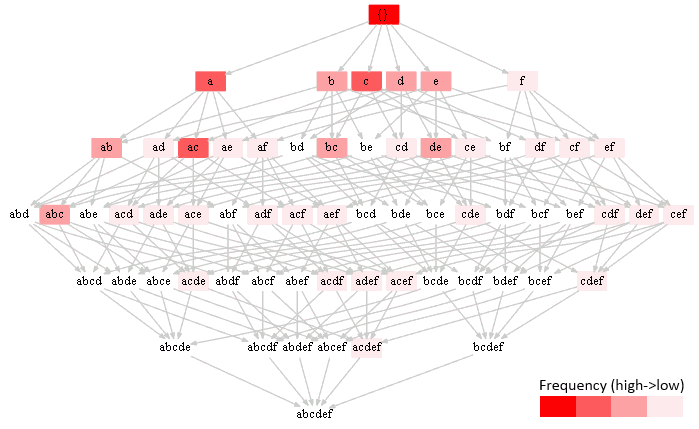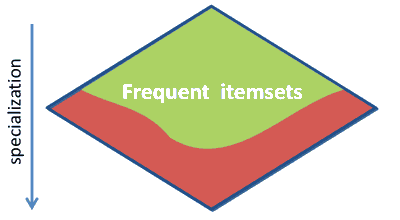ML Wiki

Frequent Pattern Mining

This is a part of Local Pattern Discovery

• that deals with Descriptive Patterns

Descriptive Patterns

Suppose that we have the following table:

Wings Beak Webfoot Fly Swim
Owl
Parrot
Flamingo
Penguin

We can say that the following are descriptive patterns

• $\text{wings}$
• $\text{beak}$
• $\text{flying}$
• $\text{wings} \land \text{beak}$
• $\text{...}$
• $\text{wings} \land \text{beak} \land \text{fly}$

They involve no rules or inference

• so they are combination of items
• they just describe things that are true with some certain probability

Frequent Patterns Mining

Goal

• finding descriptive patterns with probabilities that exceed a certain threshold

Notation

• items: set of distinct literals: $\{ a, b, c, ...\}$
• itemsets: any combination of items $\{ a, f, ... \}$
• language: all possible itemsets for the set of items
• dataset: a multiset (i.e. a set that allows duplicates) of itemsets
• an itemset is frequent if it happens more than some certain threshold

Naive Approach

• enumerate all possible itemsets
• for each possible itemset $X$ see how many occurrences there are in $D$
• i.e. calculate Support of $X$ in $F$
• if the # of occurrences is lower than some threshold, don't output it

Example

• items ${a,b,c,d,e,f}$
• 4 transactions $\big\{ \{a,b,c\}, \{a,c,d,e,f\}, \{a,b,c\}, \{d,e\} \big\}$
I = 'abcdef'
T = ['abc', 'acdef', 'abc', 'de']
for X in powerset(I):
cnt = sum([1 for i in T if set(X).issubset(set(i))])
if (cnt != 0):
print "(%s, %d)" % (''.join(X), cnt)

frequencies:

cnt itemsets
1 $f,ad,ae,af,cd,ce,cf,df,ef,acd,ace,acf,ade,adf,aef,cde,cdf,cef,def,acde,acdf,acef,adef,cdef,acdef$
2 $b,d,e,ab,bc,de,abc$
3 $a,c,ac$
4 $\{\}$

Problems:

• search space: $2^{|D|}$ - very difficult
• e.g. only 6 items - 64 combinations
• but once we found the answer for the 1st problem, we can easily find the answer for the 2nd problem

Optimization: Downward Closure

• notice that the frequency decreases when going from up to down
•• intuition: frequency can never increase when we add a new item, it can only decrease
• this is called a downward closure

Downward closure

•• If an itemset is frequent, then all its subsets are frequent.
• $X \subseteq Y, \text{supp}(Y) = t \Rightarrow \text{supp}(X) \geqslant t$
• if $abc$ occurs 3 times, then $ab$ occurs at least 3 times, but maybe more
• If an itemset is not frequent, then all its supersets are not frequent
• $X \supseteq Y, \text{supp}(X) < \text{min_t} \Rightarrow \text{supp}(Y) < \text{min_t}$
• if $abc$ occurs less than 3 times, $abcd$ also occurs less than 3 times

Algorithms

There are two ways we can traverse this lattice:

Apriori Eclat
$+$
• "Perfect" pruning of infrequent candidate itemsets
• DFS reduces memory requirements
• Usually (considerably) faster
$-$
• Can require a lot of memory (since all frequent item sets are represented)
• Support counting takes very long for large transactions
• so not always efficient in practice
• Storage of transaction lists

Association Rule Mining

After we found Frequent patterns it's easy to find association rules

• for each frequent pattern $X$ for each subset $Y \subset X$
• calculate support of $Y \to X - Y$
• if it's greater than some certain threshold, keep the rule